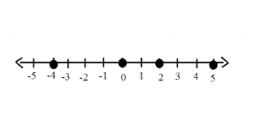# Multiply 6073

We first reduce the unknown number by 5, multiply by three and finally reduce by 4 to get the number 7. What was the unknown number?

n =  8.6667

### Step-by-step explanation:

(n-5)·3-4 = 7

3n = 26

n = 26/3 = 8.66666667

n = 26/3 ≈ 8.666667

Our simple equation calculator calculates it.Did you find an error or inaccuracy? Feel free to write us. Thank you!

Tips for related online calculators
Do you have a linear equation or system of equations and looking for its solution? Or do you have a quadratic equation?